Next: Figure 1. Static Up: NORMAL MODES OF VORTICES Previous: INTRODUCTION: VORTEX INSTABILITY

# PERTURBATION ABOUT A STATIC VORTEX

We begin by describing how the numerical diagonalization problem for the normal modes of spinwave motion about a static vortex was set up. For classical states we can parameterize the spins in terms of an in-plane angle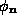and an out-of-plane angle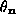, (or spin component), where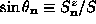, i.e.,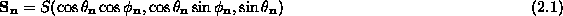For in-plane vortices, the low energy static states of this model will have all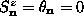, and then the equation to determine the in-plane angles becomes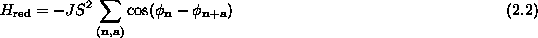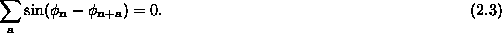Only when the vortex center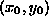is at a point of high symmetry, such as the center of a unit cell of the lattice, or directly on a lattice site (with the spin at that lattice site set perpendicular to the easy-plane) is a simple analytic solution of Eq. (2.3) known, namely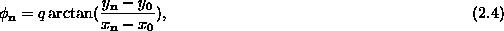where the vorticity q is an integer. For out-of-plane vortices, the in-plane angles are given by Eq. (2.4), while there are nonzero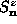components. In general, for a given value of anisotropy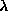, we have used a numerical relaxation procedure to determine the static vortex structure, for either in-plane or out-of-plane vortices. We start with an in-plane vortex, but perturb the spins in the unit cell containing the vortex core by giving only those sites small positive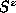components. Then every spin in the lattice is re-directed to point along the direction of the effective field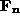due to its neighbors, as determined from the Hamiltonian above: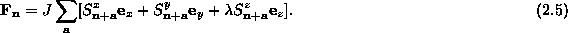The set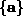includes only the displacements to the neighbors of site. Sites on the boundary of the finite system will tend to have weaker effective fields because of their smaller number of nearest neighbors in this sum. Upon iteration, this procedure relaxes the spins quickly into a static energy minimum vortex (in-plane or out-of-plane vortex) that is stable for the given value of anisotropy. This is because the spin equations of motion can be written in the form,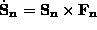; the time derivatives vanish when the spins are aligned with the effective fields of their neighbors. The relaxed vortex configuration,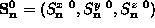can then be used to obtain the in-plane and out-of-plane angles of the static vortex, (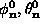), using the definition in Eq. (2.1). Some typical profiles of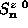so obtained for various values of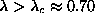for a circular system on a square lattice are shown in Fig. 1.Next: Figure 1. Static Up: NORMAL MODES OF VORTICES Previous: INTRODUCTION: VORTEX INSTABILITY

Gary M Wysin
Mon Sep 11 12:02:10 CDT 1995